# Cluster-based permutation tests on time-frequency data

## Introduction

The objective of this tutorial is to give an introduction to the statistical analysis of EEG and MEG data (denoted as M/EEG data in the following) by means of cluster-based permutation tests.

The tutorial starts with a long background section that sketches the background of permutation tests. The next sections are more tutorial-like. They deal with the analysis of an actual MEG dataset. In a step-by-step fashion, it will be shown how to statistically compare the data observed in two experimental conditions in a between-trials, in a within-trials and in a within-subjects design. For this, we will use planar TFR’s.

In this tutorial we will continue working on the dataset described in the preprocessing tutorials. Below we will repeat code to select the trials and preprocess the data as described in the earlier tutorials. We assume that the preprocessing (see the Preprocessing - Segmenting and reading trial-based EEG and MEG data tutorial), calculation of the planar gradient (see the Event-related averaging and MEG planar gradient tutorial) and the time-frequency analysis (see the Time-frequency analysis using Hanning window, multitapers and wavelets tutorial) are already clear for the reader.

This tutorial is not covering statistical test on event-related fields. If you are interested in that, you can read the Cluster-based permutation tests on event-related fields tutorial. If you are interested how parametric statistical tests can be used, you can read the Parametric and non-parametric statistics on event-related fields tutorial.

This tutorial contains hands-on material that we use for the MEG/EEG toolkit course and it is complemented by this lecture.

## Background

### The multiple comparisons problem

In the statistical analysis of MEEG-data we have to deal with the multiple comparisons problem (MCP). This problem originates from the fact that MEEG-data are multidimensional. MEEG-data have a spatiotemporal structure: the signal is sampled at multiple channels and multiple time points (as determined by the sampling frequency). The MCP arises from the fact that the effect of interest (i.e., a difference between experimental conditions) is evaluated at an extremely large number of (channel,time)-pairs. This number is usually in the order of several thousands. Now, the MCP involves that, due to the large number of statistical comparisons (one per (channel,time)-pair), it is not possible to control the so called family-wise error rate (FWER) by means of the standard statistical procedures that operate at the level of single (channel,time)-pairs. The FWER is the probability under the hypothesis of no effect of falsely concluding that there is a difference between the experimental conditions at one or more (channel,time)-pairs. A solution of the MCP requires a procedure that controls the FWER at some critical alpha-level (typically, 0.05 or 0.01). The FWER is also called the false alarm rate.

In this tutorial, we describe nonparametric statistical testing of MEEG-data. Contrary to the familiar parametric statistical framework, it is straightforward to solve the MCP in the nonparametric framework. FieldTrip contains a set of statistical functions that provide a solution for the MCP. These are the functions ft_timelockstatistics and ft_freqstatistics which compute significance probabilities using a non-parametric permutation tests. Besides nonparametric statistical tests, ft_timelockstatistics and ft_freqstatistics can also perform parametric statistical tests (see the Parametric and non-parametric statistics on event-related fields tutorial). However, in this tutorial the focus will be on non-parametric testing.

### Structure in experiment and data

#### Different types of data

MEEG-data have a spatiotemporal structure: the signal is sampled at multiple channels and multiple time points. Thus, MEEG-data are two-dimensional: channels (the spatial dimension) X time-points (the temporal dimension). In the following, these (channel,time)-pairs will be called samples. In some studies, these two-dimensional data are reduced to one-dimensional data by averaging over either a selected set of channels or a selected set of time-points.

In many studies, there is an interest in the modulation of oscillatory brain activity. Oscillatory brain activity is measured by power estimates obtained from Fourier or wavelet analyses of single-trial spatiotemporal data. Very often, power estimates are calculated for multiple data segments obtained by sliding a short time window over the complete data segment. In this way, spatio-spectral-temporal data are obtained from the raw spatiotemporal data. The spectral dimension consists of the different frequency bins for which the power is calculated. In the following, these (channel,frequency,time)-triplets will be called samples. In some studies, these three-dimensional data are reduced to one- or two-dimensional data by averaging over a selected set of channels, a selected set of time points, and/or a selected set of frequencies.

#### Structure in an experiment

The data are typically collected in different experimental conditions and the experimenter wants to know if there is a significant difference between the data observed in these conditions. To answer this question, statistical tests are used. To understand these statistical tests one has to know how the structure in the experiment affects the structure in the data. Two concepts are crucial for describing the structure in an experiment:

1. The concept of a unit of observation (UO).
2. The concept of a between- or a within-UO design. In M/EEG experiments, there are two types of UO: subjects and trials (i.e., epochs of time within a subject). Concerning the concept of a between- or a within-UO design, there are two schemes according to which the UOs can be assigned to the experimental conditions: (1) every UO is assigned to only one of a number of experimental conditions (between UO-design; independent samples), or (2) every UO is assigned to multiple experimental conditions in a particular order (within UO-design; dependent samples). Therefore, in a between-UO design, there is only one experimental condition per UO, and in a within-UO design there are multiple experimental conditions per UO. These two experimental designs require different test statistics.

The two UO-types (subjects and trials) and the two experimental designs (between- and within-UO) can be combined and this results in four different types of experiments.

### Computation of the test statistic and its significance probability

The statistical testing in FieldTrip is performed by the functions ft_timelockstatistics and ft_freqstatistics. From a statistical point of view, the calculations performed by these two functions are very similar. These functions differ with respect to the data structures on which they operate: ft_timelockstatistics operates on data structures produced by ft_timelockanalysis and ft_timelockgrandaverage, and ft_freqstatistics operates on data structures produced by ft_freqanalysis, ft_freqgrandaverage, and ft_freqdescriptives.

To correct for multiple comparisons, one has to specify the field cfg.correctm. This field can have the values ‘no’, ‘max’, ‘cluster’, ‘bonferoni’, ‘holms’, or ‘fdr’. In this tutorial, we only use cfg.correctm = ‘cluster’, which solves the MCP by calculating a so-called cluster-based test statistic and its significance probability.

#### The calculation of this cluster-based test statistic

1. For every sample (a (channel,time)-pair or a (channel,frequency,time)-triplet) the experimental conditions are compared by means of a t-value or some other number that quantifies the effect at this sample. It must be noted that this t-value is not the cluster-based test statistic for which we will calculate the significance probability; it is just an ingredient in the calculation of this cluster-based test statistic. Quantifying the effect at the sample level is possible by means of different measures. These measures are specified in cfg.statistic, which can have the values ‘ft_statfun_indepsamplesT’, ‘ft_statfun_depsamplesT’, ‘ft_statfun_actvsblT’, and many others. We will return to this in the following.
2. All samples are selected whose t-value is larger than some threshold as specified in cfg.clusteralpha. It must be noted that the value of cfg.clusteralpha does not affect the false alarm rate of the statistical test; it only sets a threshold for considering a sample as a candidate member of some cluster of samples. If cfg.clusteralpha is equal to 0.05, the t-values are thresholded at the 95-th quantile for a one-sided t-test, and at the 2.5-th and the 97.5-th quantiles for a two-sided t-test.
3. Selected samples are clustered in connected sets on the basis of temporal, spatial and spectral adjacency.
4. Cluster-level statistics are calculated by taking the sum of the t-values within every cluster.
5. The maximum of the cluster-level statistics is taken. This step and the previous one (step 4) are controlled by cfg.clusterstatistic, which can have the values ‘maxsum’, ‘maxsize’, or ‘wcm’. In this tutorial, we will only use ‘maxsum’, which is the default value. The result from step 5 is the test statistic by means of which we evaluate the effect of the experimental conditions.

#### The calculation of the significance probability

If the MCP is solved by choosing for a cluster-based test statistic (i.e., with cfg.correctm = ‘cluster’), the significance probability can only be calculated by means of the so-called Monte Carlo method. This is because the reference distribution for a permutation test can be approximated (to any degree of accuracy) by means of the Monte Carlo method. In the configuration, the Monte Carlo method is chosen as follows: cfg.method = ‘montecarlo’.

The Monte Carlo significance probability is calculated differently for a within- and between-UO design. We now show how the Monte Carlo significance probability is calculated for in a between-trials experiment (a between-UO design with trials as UO

1. Collect the trials of the different experimental conditions in a single set.
2. Randomly draw as many trials from this combined data set as there were trials in condition 1 and place them into subset 1. The remaining trials are placed in subset 2. The result of this procedure is called a random partition.
3. Calculate the test statistic (i.e., the maximum of the cluster-level summed t-values) on this random partition.
4. Repeat steps 2 and 3 a large number of times and construct a histogram of the test statistics. The computation of this Monte Carlo approximation involves a user-specified number of random draws (specified in cfg.numrandomization). The accuracy of the Monte Carlo approximation can be increased by choosing a larger value for cfg.numrandomization.
5. From the test statistic that was actually observed and the histogram in step 4, calculate the proportion of random partitions that resulted in a larger test statistic than the observed one. This proportion is the Monte Carlo significance probability, which is also called a p-value.

If the p-value is smaller than the critical alpha-level (typically 0.05) then we conclude that the data in the two experimental conditions are significantly different. This p-value can also be calculated for the second largest cluster-level statistic, the third largest, etc. It is common practice to say that a cluster is significant if its p-value is less than the critical alpha-level. This practice suggests that it is possible to do spatio-spectral-temporal localization by means of the cluster-based permutation test. However, from a statistical perspective, this suggestion is not justified. Consult Maris and Oostenveld, Journal of Neuroscience Methods, 2007 for an elaboration on this topic.

## Procedure

In this tutorial we will consider a between-trials experiment, in which we analyze the data of a single subject. For the statistical analysis for this experiment we calculate the planar TFRs. The steps we perform are as follow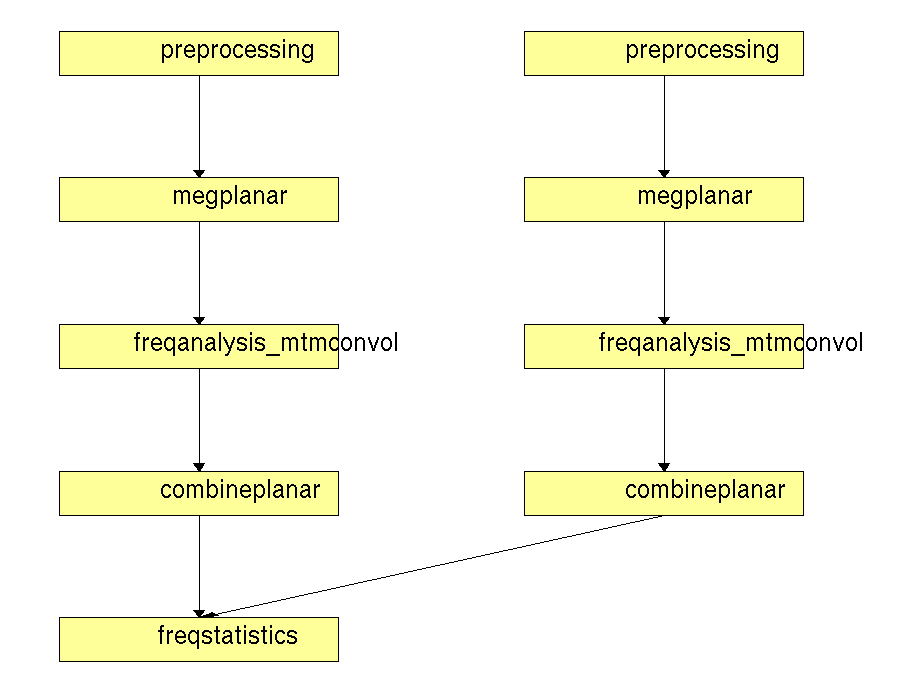Figure 1. Pipeline of statistical analysis of planar TFR’s in a between trials design

Subsequently we will consider a within-trials experiment, in which we compare the pre-stimulus baseline to the post-stimulus activity time window. The steps we perform are as follows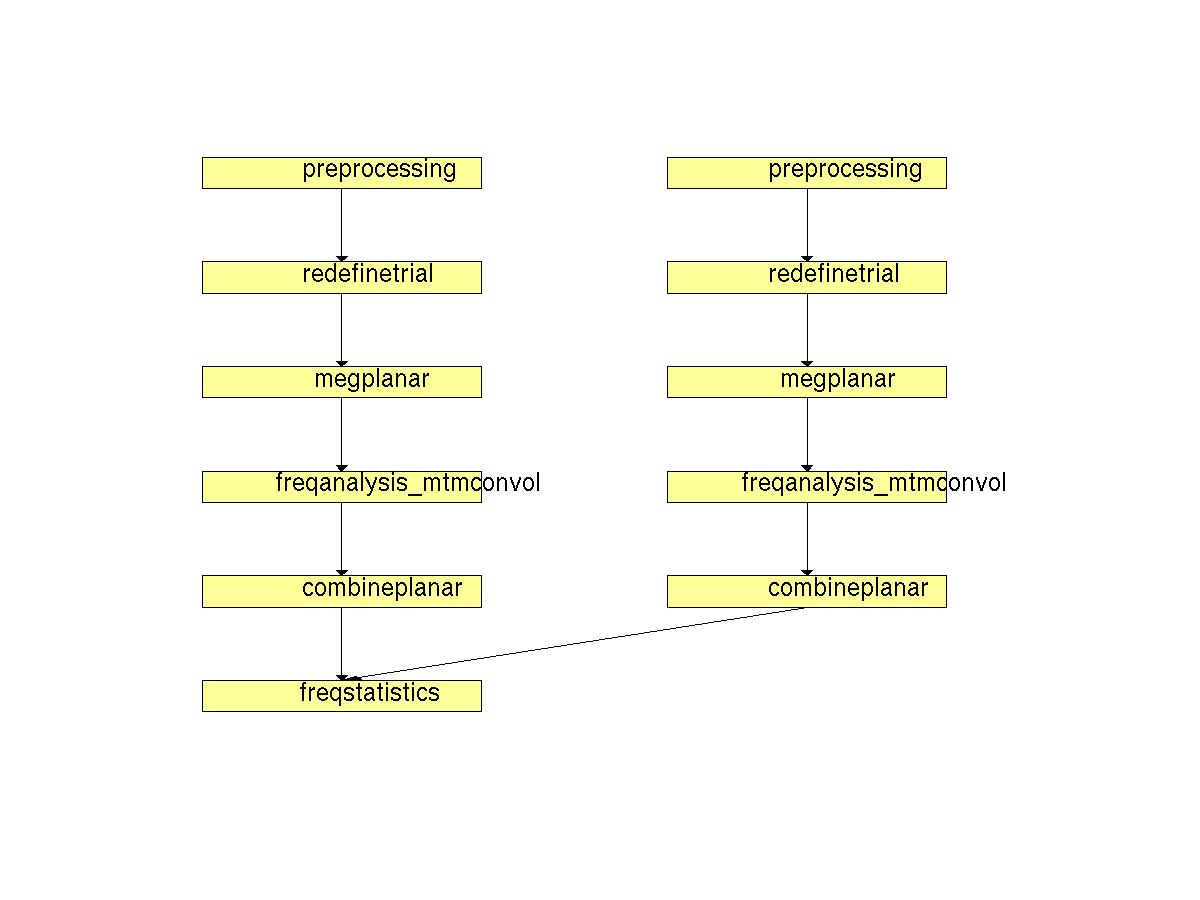Figure 2. Pipeline of statistical analysis of planar TFR’s in a within-trials design

Finally we will consider a within-subjects experiment with the following step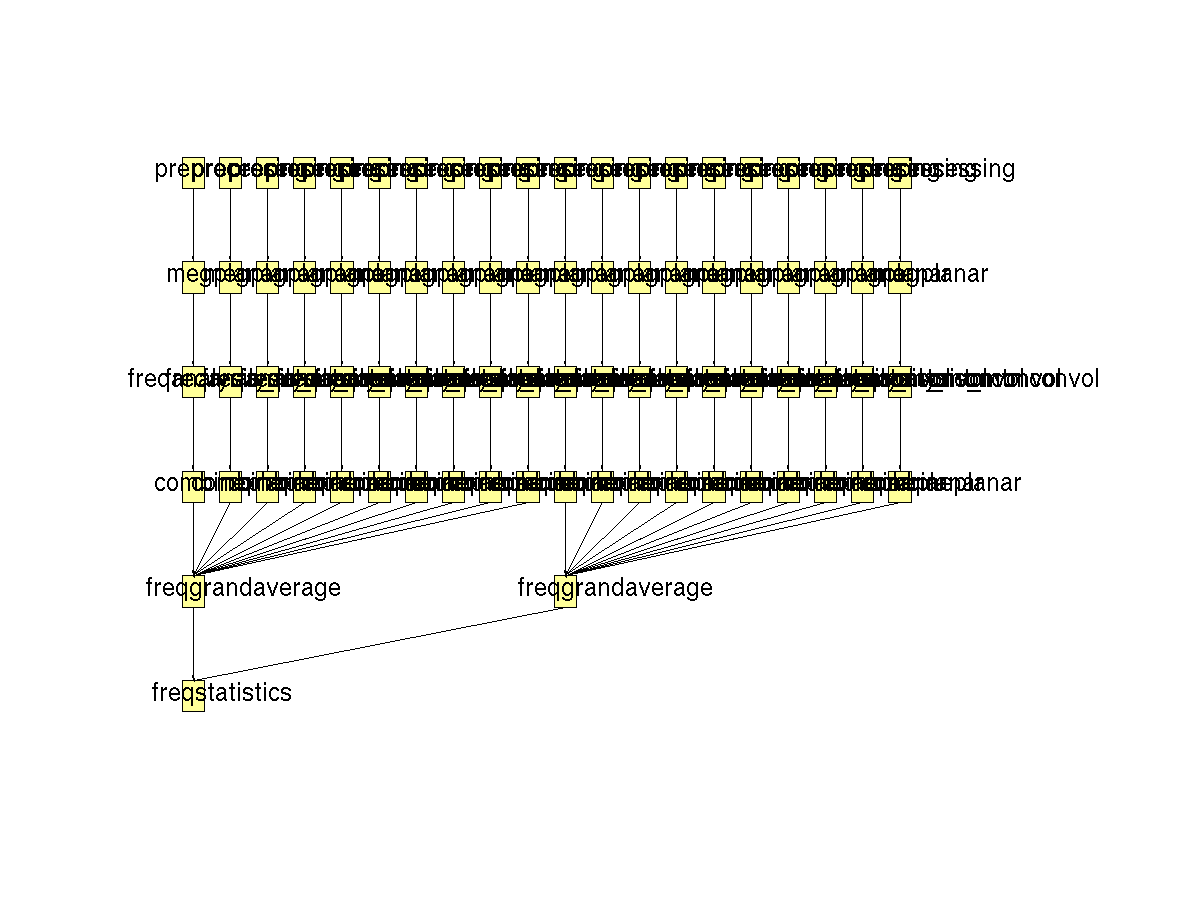Figure 3. Pipeline of statistical analysis of planar TFR’s in a within-subjects design

## Between-trial experiments

In a between-trials experiment, we analyze the data of a single subject. By means of a statistical test, we want to answer the question whether there is a systematic difference in the MEG recorded on trials with a fully congruent and trials with a fully incongruent sentence ending.

### Preprocessing

We will now read and preprocess the data. If you would like to continue directly with the already preprocessed data, you can download dataFIC.mat and dataFC.mat. Load the data into MATLAB with the command `load` and skip to Calculation of the planar gradient and time-frequency analysis.

Otherwise run the following code:

The ft_definetrial and ft_preprocessing functions require the original MEG dataset, which is available here

Do the trial definition for the all conditions together:

``````cfg                         = [];
cfg.dataset                 = 'Subject01.ds';
cfg.trialfun                = 'ft_trialfun_general'; % this is the default
cfg.trialdef.eventtype      = 'backpanel trigger';
cfg.trialdef.eventvalue     = [3 5 9]; % the values of the stimulus trigger for the three conditions
% 3 = fully incongruent (FIC), 5 = initially congruent (IC), 9 = fully congruent (FC)
cfg.trialdef.prestim        = 1; % in seconds
cfg.trialdef.poststim       = 2; % in seconds

cfg = ft_definetrial(cfg);
``````

### Cleaning

``````% remove the trials that have artifacts from the trl
cfg.trl([2, 5, 6, 8, 9, 10, 12, 39, 43, 46, 49, 52, 58, 84, 102, 107, 114, 115, 116, 119, 121, 123, 126, 127, 128, 133, 137, 143, 144, 147, 149, 158, 181, 229, 230, 233, 241, 243, 245, 250, 254, 260],:) = [];

% preprocess the data
cfg.channel   = {'MEG', '-MLP31', '-MLO12'};        % read all MEG channels except MLP31 and MLO12
cfg.demean    = 'yes';                              % do baseline correction with the complete trial

data_all = ft_preprocessing(cfg);
``````

These data have been cleaned from artifacts by removing several trials and two sensors; see the visual artifact rejection tutorial.

For subsequent analysis we extract the trials of the fully incongruent condition and the fully congruent condition to separate data structures.

``````cfg = [];
cfg.trials = data_all.trialinfo == 3;
dataFIC = ft_redefinetrial(cfg, data_all);

cfg = [];
cfg.trials = data_all.trialinfo == 9;
dataFC = ft_redefinetrial(cfg, data_all);
``````

Subsequently you can save the data to disk.

``````save dataFIC dataFIC
save dataFC dataFC
``````

### Calculation of the planar gradient and time-frequency analysis

Before calculating the TFRs we calculate the planar gradient with ft_megplanar. This requires preprocessed data (see above or download dataFIC.mat and dataFC.mat).

``````load dataFIC

cfg = [];
cfg.planarmethod = 'sincos';
% prepare_neighbours determines with what sensors the planar gradient is computed
cfg_neighb.method = 'distance';
cfg.neighbours    = ft_prepare_neighbours(cfg_neighb, dataFC);

dataFIC_planar = ft_megplanar(cfg, dataFIC);
dataFC_planar  = ft_megplanar(cfg, dataFC);
``````

Without a prior hypothesis (i.e., an hypothesis that is independent of the present data), we must test the difference between the FIC and the FC condition for all frequency bands that may be of interest. However, such an analysis is not suited as a first step in a tutorial. Therefore, we assume that we have a prior hypothesis about the frequency band in which the FIC and FC condition may differ. This frequency band is centered at 20 Hz. We will now investigate if there is a difference between the FIC and the FC condition at this frequency. To calculate the TFRs, we use ft_freqanalysis with the configuration below. See the tutorial Time-frequency analysis using Hanning window, multitapers and wavelets for an explanation of the settings. Note that cfg.keeptrials = ‘yes’, which is necessary for the subsequent statistical analysis.

``````cfg = [];
cfg.output     = 'pow';
cfg.channel    = 'MEG';
cfg.method     = 'mtmconvol';
cfg.taper      = 'hanning';
cfg.foi        = 20;
cfg.toi        = [-1:0.05:2.0];
cfg.t_ftimwin  = 7./cfg.foi; %7 cycles
cfg.keeptrials = 'yes';
``````

Calculate the TFRs for the two experimental conditions (FIC and FC).

``````freqFIC_planar = ft_freqanalysis(cfg, dataFIC_planar);
freqFC_planar  = ft_freqanalysis(cfg, dataFC_planar);
``````

Finally, we calculate the combined planar gradient and copy the gradiometer structure in the new datasets.

``````cfg = [];
freqFIC_planar_cmb = ft_combineplanar(cfg, freqFIC_planar);
freqFC_planar_cmb = ft_combineplanar(cfg, freqFC_planar);

``````

To save:

``````save freqFIC_planar_cmb freqFIC_planar_cmb
save freqFC_planar_cmb  freqFC_planar_cmb
``````

### Permutation test

Now, run ft_freqstatistics to compare freqFIC_planar_cmb and freqFC_planar_cmb. Except for the field cfg.latency, the following configuration is identical to the configuration that was used for comparing event-related averages in the Cluster-based permutation tests on event-related fields tutorial. Also see this tutorial for a detailed explanation of all the configuration settings. You can read more about the ft_prepare_neighbours function in the FAQs.

``````load freqFIC_planar_cmb

cfg = [];
cfg.channel          = {'MEG', '-MLP31', '-MLO12'};
cfg.latency          = 'all';
cfg.frequency        = 20;
cfg.method           = 'montecarlo';
cfg.statistic        = 'ft_statfun_indepsamplesT';
cfg.correctm         = 'cluster';
cfg.clusteralpha     = 0.05;
cfg.clusterstatistic = 'maxsum';
cfg.minnbchan        = 2;
cfg.tail             = 0;
cfg.clustertail      = 0;
cfg.alpha            = 0.025;
cfg.numrandomization = 500;
% prepare_neighbours determines what sensors may form clusters
cfg_neighb.method    = 'distance';
cfg.neighbours       = ft_prepare_neighbours(cfg_neighb, dataFC);

design = zeros(1,size(freqFIC_planar_cmb.powspctrm,1) + size(freqFC_planar_cmb.powspctrm,1));
design(1,1:size(freqFIC_planar_cmb.powspctrm,1)) = 1;
design(1,(size(freqFIC_planar_cmb.powspctrm,1)+1):(size(freqFIC_planar_cmb.powspctrm,1)+...
size(freqFC_planar_cmb.powspctrm,1))) = 2;

cfg.design           = design;
cfg.ivar             = 1;

[stat] = ft_freqstatistics(cfg, freqFIC_planar_cmb, freqFC_planar_cmb);
``````

Save the output:

``````save stat_freq_planar_FICvsFC stat
``````

### Plotting the results

The output can also be obtained from stat_freq_planar_FICvsFC.mat. If you need to reload the statistics output, us

``````load stat_freq_planar_FICvsFC
``````

By inspecting stat.posclusters and stat.negclusters, you will see that there is one large cluster that shows a negative effect and no large clusters showing a positive effect.

To show the topography of the negative cluster, we make use of ft_clusterplot. This is a function that displays the channels that contribute to large clusters, based on which the null-hypothesis can be rejected. First we use ft_freqdescriptives to average over the trials.

``````cfg = [];
freqFIC_planar_cmb = ft_freqdescriptives(cfg, freqFIC_planar_cmb);
freqFC_planar_cmb  = ft_freqdescriptives(cfg, freqFC_planar_cmb);
``````

Subsequently we add the raw effect (FIC-FC) to the obtained stat structure and plot the largest cluster overlayed on the raw effect.

``````stat.raweffect = freqFIC_planar_cmb.powspctrm - freqFC_planar_cmb.powspctrm;

cfg = [];
cfg.alpha  = 0.025;
cfg.parameter = 'raweffect';
cfg.zlim   = [-1e-27 1e-27];
cfg.layout = 'CTF151_helmet.mat';
ft_clusterplot(cfg, stat);
``````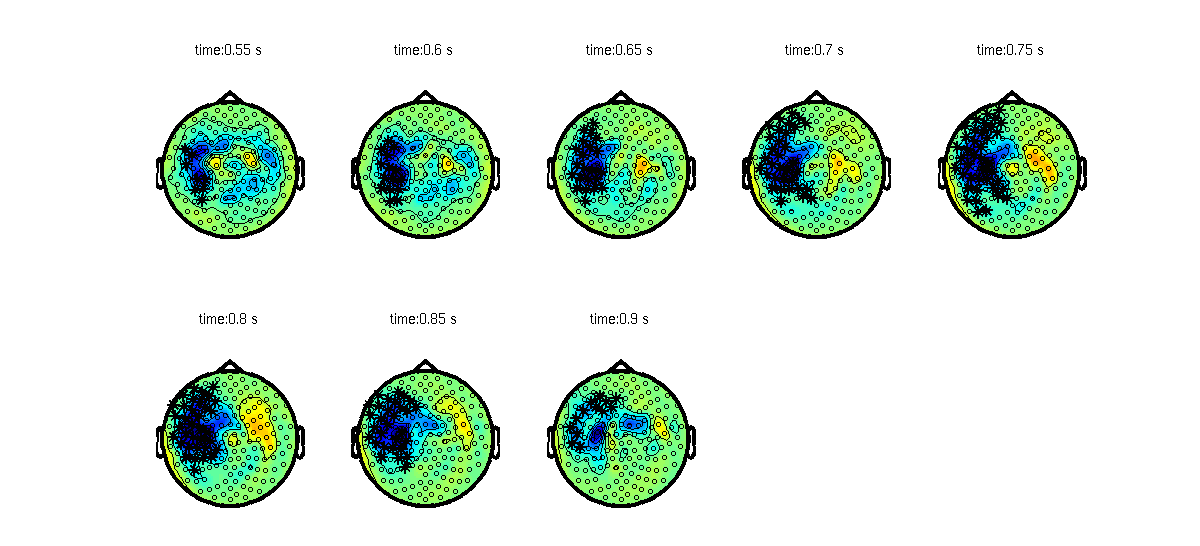Figure 1: Raw effect (FIC-FC) and channel-time cluster of planar gradient TFRs of subject 1.

## Within trial experiments

We will now show how to statistically test the difference between the TFRs in the post-stimulus (activation) and the pre-stimulus (baseline) period of the fully incongruent sentence endings. To perform this comparison by means of a permutation test, we have to select equal-length non-overlapping time intervals in the activation and the baseline period. For the activation period we choose [0.6 1.6], which is the time interval from 0.6 to 1.6 seconds after stimulus onset. and for the baseline period we choose [-1 0], which is the time interval from 1 to 0 seconds before stimulus onset.

It must be stressed that the time windows we choose to compare are nonoverlapping and of equal length. This constraint follows from the null hypothesis that is tested with a permutation test. This null hypothesis involves that the data (spatiotemporal matrices) observed in the two experimental conditions are drawn from the same probability distribution. This null hypothesis only makes sense if the dimensions of the data matrices in the two experimental conditions are equal. In other words, the number of channels and the number of time points of these spatiotemporal data matrices must be equal. This also applies to a within trials experiment in which an activation and a baseline condition are compared: the number of channels and time points of the two data matrices must be equal.

### Preprocessing and freqanalysis on planar data

We first select equal-length non-overlapping time intervals in the activation and the baseline period. For the activation period we choose [0.6 1.6], the time interval from 0.6 to 1.6 seconds after stimulus onset. We will again focus on the power in the beta band (around 20 Hz). And for the baseline period we choose [-1 0], the time interval from 1 to 0 seconds before stimulus onset.

We now calculate the TFRs for the activation and the baseline period. We use again the preprocessed dataFIC (see above or download dataFIC.mat).

``````load dataFIC
``````

To calculate the TFRs with the planar gradient, we first cut out the time intervals of interest with the function ft_redefinetrial.

``````cfg = [];
cfg.toilim = [0.6 1.6];
dataFIC_activation = ft_redefinetrial(cfg, dataFIC);

cfg = [];
cfg.toilim = [-1.0 0];
dataFIC_baseline = ft_redefinetrial(cfg, dataFIC);
``````

ft_freqstatistics will always compare data at the same time point, and therefore you get an error if you try to statistically test differences between data structures with non-overlapping time axes. This implies that, in order to statistically test the differences between activation and baseline period data, we have to trick the function by making the time axis the same. We do this by copying the time axis of dataFIC_activation onto dataFIC_baseline.

``````dataFIC_baseline.time = dataFIC_activation.time;
``````

To calculate the TFRs for the synthetic planar gradient data, we must run the following code:

``````cfg = [];
cfg.planarmethod = 'sincos';
% prepare_neighbours determines with what sensors the planar gradient is computed
cfg_neighb.method    = 'distance';
cfg.neighbours       = ft_prepare_neighbours(cfg_neighb, dataFIC_activation);
dataFIC_activation_planar = ft_megplanar(cfg, dataFIC_activation);
dataFIC_baseline_planar   = ft_megplanar(cfg, dataFIC_baseline);

cfg = [];
cfg.output = 'pow';
cfg.channel = 'MEG';
cfg.method = 'mtmconvol';
cfg.taper = 'hanning';
cfg.foi = 20;
cfg.toi = [0.6:0.05:1.6];
cfg.t_ftimwin = 7./cfg.foi; %7 cycles
cfg.keeptrials = 'yes';

freqFIC_activation_planar = ft_freqanalysis(cfg, dataFIC_activation_planar);
freqFIC_baseline_planar   = ft_freqanalysis(cfg, dataFIC_baseline_planar);
``````

``````cfg = [];
freqFIC_baseline_planar_cmb   = ft_combineplanar(cfg,freqFIC_baseline_planar);
freqFIC_activation_planar_cmb = ft_combineplanar(cfg,freqFIC_activation_planar);

``````

To save:

``````save freqFIC_baseline_planar_cmb freqFIC_baseline_planar_cmb;
save freqFIC_activation_planar_cmb freqFIC_activation_planar_cmb;
``````

### Permutation test

``````load freqFIC_activation_planar_cmb
``````

To compare `freqFIC_activation_planar_cmb` and `freqFIC_baseline_planar_cmb` by means of ft_freqstatistics, we use the following configuration:

``````cfg = [];
cfg.channel          = {'MEG', '-MLP31', '-MLO12'};
cfg.latency          = [0.8 1.4];
cfg.method           = 'montecarlo';
cfg.frequency        = 20;
cfg.statistic        = 'ft_statfun_actvsblT';
cfg.correctm         = 'cluster';
cfg.clusteralpha     = 0.05;
cfg.clusterstatistic = 'maxsum';
cfg.minnbchan        = 2;
cfg.tail             = 0;
cfg.clustertail      = 0;
cfg.alpha            = 0.025;
cfg.numrandomization = 500;
% prepare_neighbours determines what sensors may form clusters
cfg_neighb.method    = 'distance';
cfg.neighbours       = ft_prepare_neighbours(cfg_neighb, freqFIC_activation_planar_cmb);

ntrials = size(freqFIC_activation_planar_cmb.powspctrm,1);
design  = zeros(2,2*ntrials);
design(1,1:ntrials) = 1;
design(1,ntrials+1:2*ntrials) = 2;
design(2,1:ntrials) = [1:ntrials];
design(2,ntrials+1:2*ntrials) = [1:ntrials];

cfg.design   = design;
cfg.ivar     = 1;
cfg.uvar     = 2;
``````

This configuration for a within-trials experiment is very similar to the configuration for the within-subjects experiment in the “Cluster-based permutation tests on event-related fields” tutorial in which we compared the evoked responses to fully incongruent and fully congruent sentence endings. The main difference is the measure that we use to evaluate the effect at the sample level (cfg.statistic = ‘ft_statfun_actvsblT’ instead of cfg.statistic = ‘ft_statfun_depsamplesT’). With cfg.statistic = ‘ft_statfun_actvsblT’, we choose the so-called activation-versus-baseline T-statistic. This statistic compares the power in every sample (i.e., a (channel,frequency,time)-triplet) in the activation period with the corresponding time-averaged power (i.e., the average over the temporal dimension) in the baseline period. The comparison of the activation and the time-averaged baseline power is performed by means of a dependent samples T-statistic.

We can now run ft_freqstatistics

``````[stat] = ft_freqstatistics(cfg, freqFIC_activation_planar_cmb, freqFIC_baseline_planar_cmb);
``````

Save the output:

``````save stat_freqFIC_ACTvsBL stat
``````

The output can also be obtained from stat_freqFIC_ACTvsBL.mat. If you need to reload the statistics output, use:

``````load stat_freqFIC_ACTvsBL
``````

By inspecting stat.posclusters and stat.negclusters, you will see that there is one substantially large cluster showing a positive effect and no large clusters showing a negative effect.

### Plotting the results

This time we will plot the largest cluster on top of the statistics, which are present in the .stat field.

``````cfg = [];
cfg.alpha     = 0.025;
cfg.parameter = 'stat';
cfg.zlim      = [-4 4];
cfg.layout    = 'CTF151_helmet.mat';
ft_clusterplot(cfg, stat);
``````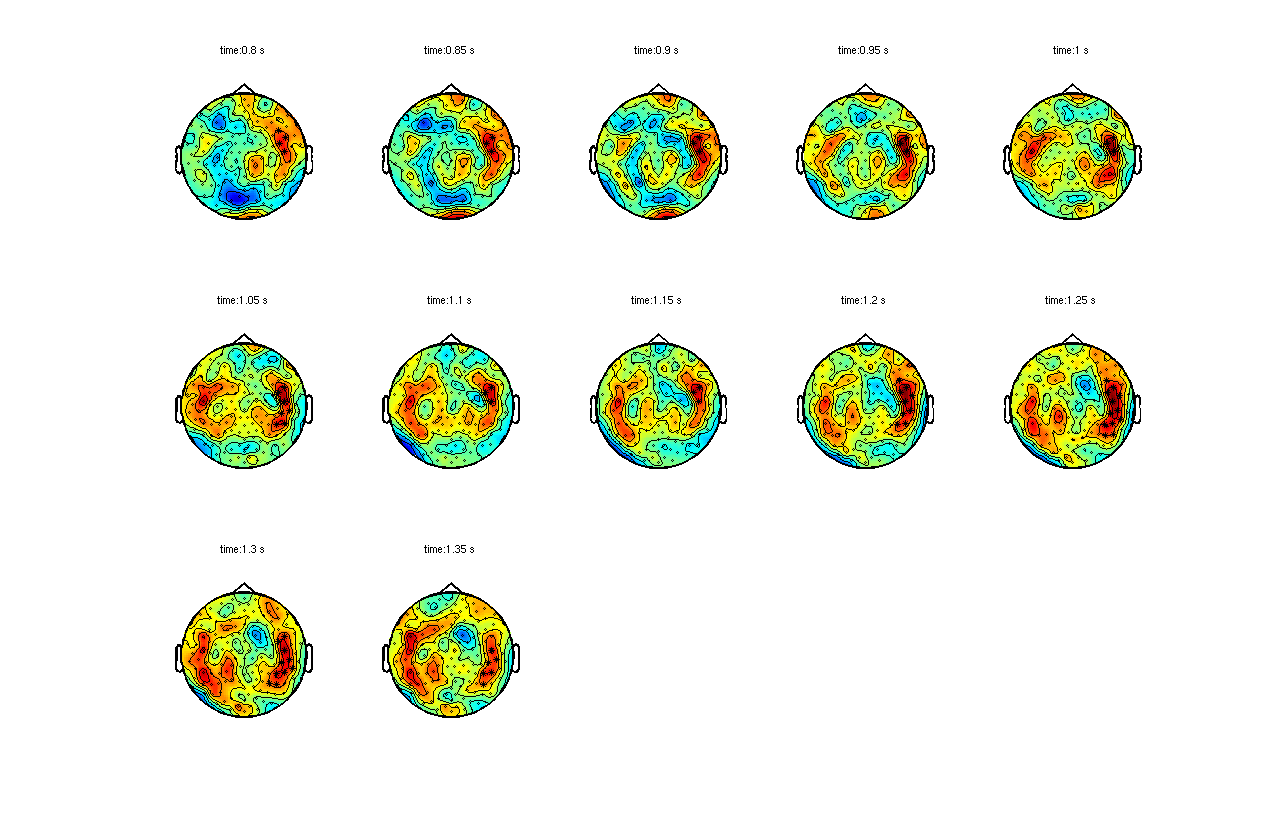Figure 2: Largest cluster that shows a difference between activation and baseline, plotted on top of the T-statistic of the difference..

## Within subjects experiments

In this paragraph we describe permutation testing for TFRs obtained in experiments involving multiple subjects that are each observed in multiple experimental conditions. Every subject is observed in a large number of trials, each one belonging to one experimental condition. For every subject, averages are computed over all trials belonging to each of the experimental conditions. Thus, for every subject, the data are summarized in an array of condition-specific averages. The permutation test that will be described in the following informs us about the following null hypothesis: the probability distribution of the condition-specific averages is identical for all experimental conditions.

### Preprocessing, planar gradient and grand average

To test the difference between the average TFRs for fully incongruent (FIC) and fully congruent (FC) sentence endings we use planar gradient data. To load the data structures containing time frequency grand averages of all ten subjects (available here), use:

``````load GA_TFR_orig;
``````

The averages of the TFRs for the fully incongruent and the fully congruent sentence endings are stored in GA_TFRFIC and GA_TFRFC. These averages were calculated using ft_freqgrandaverage .

### Permutation test

We now perform the permutation test using ft_freqstatistics. The configuration setting for this analysis are almost identical to the settings for the within-subjects experiment in the “Cluster-based permutation tests on event-related fields” tutorial. The only difference is a small change in the latency window (cfg.latency). You can read more about the ft_prepare_neighbours function in the FAQs.

``````cfg = [];
cfg.channel          = {'MEG'};
cfg.latency          = [0 1.8];
cfg.frequency        = 20;
cfg.method           = 'montecarlo';
cfg.statistic        = 'ft_statfun_depsamplesT';
cfg.correctm         = 'cluster';
cfg.clusteralpha     = 0.05;
cfg.clusterstatistic = 'maxsum';
cfg.minnbchan        = 2;
cfg.tail             = 0;
cfg.clustertail      = 0;
cfg.alpha            = 0.025;
cfg.numrandomization = 500;
% specifies with which sensors other sensors can form clusters
cfg_neighb.method    = 'distance';
cfg.neighbours       = ft_prepare_neighbours(cfg_neighb, GA_TFRFC);

subj = 10;
design = zeros(2,2*subj);
for i = 1:subj
design(1,i) = i;
end
for i = 1:subj
design(1,subj+i) = i;
end
design(2,1:subj)        = 1;
design(2,subj+1:2*subj) = 2;

cfg.design   = design;
cfg.uvar     = 1;
cfg.ivar     = 2;

[stat] = ft_freqstatistics(cfg, GA_TFRFIC, GA_TFRFC)
``````

Save the output:

``````save stat_freq_planar_FICvsFC_GA stat
``````

The output can also be obtained from stat_freq_planar_FICvsFC_GA.mat. If you need to reload the statistics output, us

``````load stat_freq_planar_FICvsFC_GA
``````

From inspection of stat.posclusters and stat.negclusters, we observe that there is one significant negative cluster.

### Plotting the results

Plot again with ft_clusterplot:

``````cfg = [];
cfg.alpha  = 0.025;
cfg.parameter = 'stat';
cfg.zlim   = [-4 4];
cfg.layout = 'CTF151_helmet.mat';
ft_clusterplot(cfg, stat);
``````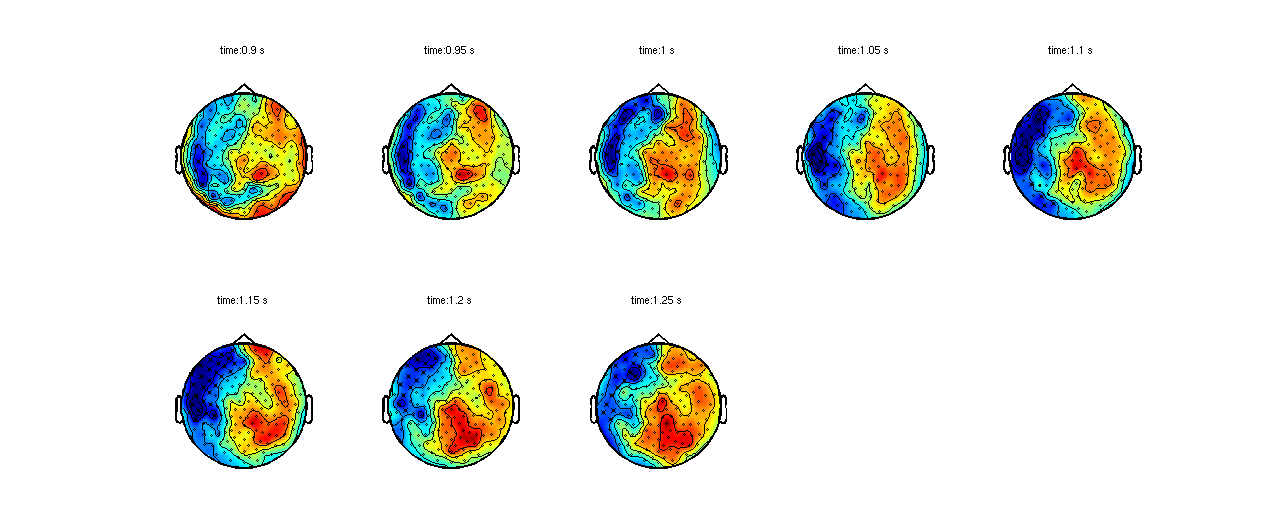Figure 3: T-statistic of the difference (FIC-FC) (of the combined planar gradient TFRs) and largest channel-time clusters.

#### Exercise

Try calling clusterplot with cfg.alpha = 0.05;

## Summary and suggested further readings

In this tutorial, we showed how to do non-parametric statistical test, cluster-based permutation test on planar TFR’s with between-trials, with within-trials and with within-subjects experimental designs. It was also shown how to plot the results.

If you are interested in parametric tests, you can read the Parametric and non-parametric statistics on event-related fields tutorial. If you are interested in how to do the same statistics on event-related fields, you can read the Cluster-based permutation tests on event-related fields tutorial.

If you would like to read more about statistical analysis, you can look at the following FAQs:

and example scripts: